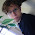## 2012-05-04

### Best Designs - Linac900Ext10tc2

We come to the end of an era, with 10tc2. This lattice, released in July 2011, marked the last of the v4.44 compatable lattices, and the end of the line for the v4.44x clients that have been going since 2007.

The best design was 3.774999% by a Boinc Wrapper user (there is no way to tell who it is)

v4.45 had been out for some time, but not everyone had upgraded. This was especially true for the BOINC wrapper users, and to give them time to migrate, this last lattice was released. It also gave us one last chance to test out the issues of RF phase and optomisation, and allowing a for-like comparison with 10td2.

The change in optimisation pathway gave an improved result, scoring 0.642961% better over the 10td2 lattice.

The search space is in the 10354 range with 118 parameters. The lattice showed a two-stage growth pattern.

This is the 'best' design as recreated in v4.45. The original simulation length as recorded was 1 hour, 30 minutes 06 seconds.

Music is Trip to STatos by cyberjeff released under a CC-BY-NC-SA license

Next time, Linac900Ext9XDATA
Lattice
Linac900Ext10td2
Date of release
July 12, 2011
Date Ended
February 10, 2012
Total possible number of permutations
10354 (1 with 354 zeros after it)
Number of Simulations Run
1,533,702
Total MPTS
2,791,778,549.4
Target Energy Range
830.41MeV - 969.64MeV
Design

tantalumrodr=000;tantalumrodz=351;d1l=079;d2l=000;d3l=831;d4l=480;d5l=441;db10l=805;db11l=296;db1l=000;db2l=999;db3l=763;db4l=998;db5l=992;db6l=846;db7l=914;db8l=697;db9l=835;decaycells=020;ld1=000;ld10=262;ld2=000;ld3=144;ld4=177;ld5=127;ld6=000;ld7=000;ld8=865;ld9=997;linaccells=998;ls10f=998;ls10l=841;ls1f=002;ls1l=996;ls2f=020;ls2l=998;ls3f=011;ls3l=999;ls4f=000;ls4l=884;ls5f=011;ls5l=916;ls6f=998;ls6l=609;ls7f=996;ls7l=612;ls8f=000;ls8l=978;ls9f=000;ls9l=999;rf10p=465;rf10v=926;rf1p=998;rf1v=999;rf2p=029;rf2v=994;rf3p=052;rf3v=999;rf4p=049;rf4v=067;rf5p=559;rf5v=216;rf6p=000;rf6v=000;rf7p=024;rf7v=999;rf8p=000;rf8v=000;rf9p=758;rf9v=988;s1f=999;s1l=984;s2f=999;s2l=991;s2r=345;s3f=996;s3l=998;s3r=626;s4f=505;s4l=433;s4r=993;s5f=193;s5l=605;s5r=936;s6f=428;s6l=999;s6r=999;sb10f=498;sb10l=537;sb10r=591;sb1f=991;sb1l=998;sb1r=000;sb2f=998;sb2l=056;sb2r=744;sb3f=000;sb3l=986;sb3r=413;sb4f=230;sb4l=998;sb4r=296;sb5f=763;sb5l=973;sb5r=137;sb6f=260;sb6l=998;sb6r=899;sb7f=507;sb7l=569;sb7r=377;sb8f=597;sb8l=813;sb8r=289;sb9f=680;sb9l=878;sb9r=357;#gen=6;#runs=5;
3.774999 (14317.5 Mpts) [v4.44d] <Linac900Ext10td2> by boinc (Boinc Wrapper)

#### 1 comment:

1.And typically, as I posted the links to the muon1 site, I noticed that a few hours ago, a better result was submitted. Alas, these videos are done on Wednesdays, and everything was already done. However, I will note that [DPC] Team ColdFusion~Arnim did submit a better score, of 3.775458%, today.

This is why late flushes are bad. Alas, he missed his second video (his first was PhaseRotDD) by waiting too long to submit.